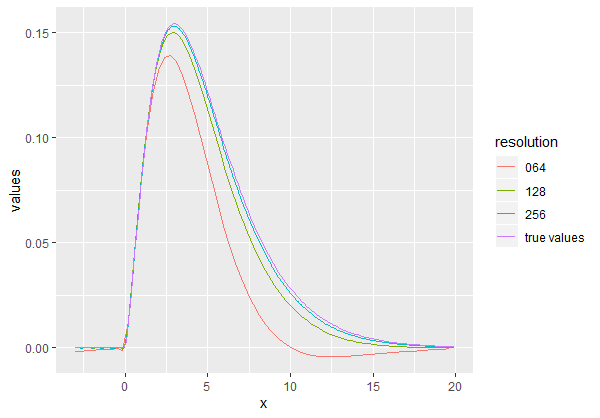# mathclasstutor

Mathematics, Science, statistics, economics, finance, econometrics, Business Mathematics, statistical analysis, R language, Computer Science, Stock Analysis, APPLE Stock Analysis, Amazon stock Analysis, GARCH model, Arch model,q-q plot,ols, regression, Time Series, t-test, cofactor, variance, standard deviation,Matlab,Nifty 50 Analysis,BMW stock analysis,

## Email Subscribers

Delivered by FeedBurner

## The logit is the log-odds of the probability. We have seen the idea of a fitting straight line but have not encountered a constraint on the fitted models, such as p always remaining between 0 and 1, as in this case. The solution is to introduce a new class of models. We define the logit of p as the logarithm(base e) of the odds.Mathematically, logit (p)= log(p/(1-p)). The ratio p/(1-p) is the odds or ratio of the probability of the event to the probability of the complementary event. The odds ratio is familiar to epidemiologists and horse-race handicappers alike. The logist is the log-odds of an event occurring. In epidemiology, we usually talk about the odds rather than the probability of an event , especially when the events are rare.                                                      It does not matter much which event is considered a "success" or" failure" in the binomial distribution. Recall the property of the logarithm of a reciprocal for any positive number z.   log(1/z)= -log(z)                                                                                               so we have logit (p)= log(p/(1-p))= -logit(1-p)

This is, the logit of 'success' is the negative of the logit of "failure". We only need to remember which outcome we are calling a "success". The logit is the log-odds of the probability.                                                                                                                                                  Let us take a moment to motivate to use of the logit. The p parameter is restricted to values between 0 and 1. The logit transforms p to cover the entire number line.A plot of logit (p) against p is given in the figure.From this figure we see how values o p are spread out from the interval of 0 to 1, this transformation takes of extremely large negative and positive values, respectively.                                                                            From this figure we can see that logit (p) is 0 when p is 1/2.similarly, logit(p) is negative when p is less than 1/2. The plot of logit(1-p) in this figure demonstrates the relation given at equation, the logit of "failure" is the negative  of the logit of "success"
.

">